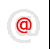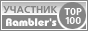Apache-Talk @lexa.ruInet-Admins @info.east.ruSecurity-alerts @yandex-team.runginx-ru @sysoev.ruđéűéôĺđéóříááňčé÷ :: Filmscanners
Filmscanners mailing list archive (filmscanners@halftone.co.uk)

### RE: filmscanners: New auto adjust software on it's way

```> Yeah, that article has stuck in my mind also. I remember
> that the method had to do with mathematically analyzing
> circles of confusion to sharpen unsharp images (don't
> remember anything about motion blur, but it might have been
> there). I onced asked about it on some list or other and
> someone mentioned that there was some problem or other. Be
> curious if anyone has any less vague info about the
> technique.
>
> John M.
>

WARNING: science and math explanation - please skip if not interested

A simple way of looking at the image formed by an optical system to consider
the image to be the convolution between the ideal image and the point spread
function for the optics.  The ideal image is just the image that you would
have if the optics had no aberrations and light did not diffract.  The point
spread function is the real image that would form if you were looking at a
point source, such as a star.  The convolution is a two dimensional
integration that combines the point spread function with each point in the
ideal image.

If the ideal image consists of just one star, the real image would be a
single point spread function properly located and with the correct
intensity.  Add another star to the ideal image and add the corresponding
point spread function to the real image.  Keep adding points to the ideal
image until it looks like the scene you are photographing and add the
corresponding point spread function to the real image.  If the array of
points looks sufficiently close the input scene the real image (smeared by
the point spread function) will be what finally reaches the film.

Various complications arise.  To model an image of a colored object the
above process needs to be repeated for the different colors.  If the point
spread function varies across the image (as it would for any real optics)
then the appropriate point spread function must be used.

Instead of convolving the ideal image with the point spread function, the
mathematically equivalent operation can be carried out using Fourier
transforms.  Instead of doing a two dimensional integration one Fourier
transforms both the ideal image and the point spread function, multiplies
them together, and then performs an inverse Fourier Transforms. The end
result is the same smeared image the convolution obtained.

Long ago it was realized that doing the math using Fourier transforms was
both quicker and it would allow the smearing to be undone, at least in
principle.  If the smeared image is just the product of the point spread
function and the ideal image, then the ideal image should be the smeared
imaged divided by the point spread function.  There are MAJOR problems with
this simple minded approach, but it is the basis of most current imagine
sharpening techniques.  Some of the problems include dividing by zero, noisy

Various methods have been proposed to overcome the problems, but only a
small class of images are practical to sharpen.  Stellar images from the
Huble telescope sharpen very nicely.  Images of grandma taken with a cheap
zoom lens are a lost cause.

```Copyright © Lexa Software, 1996-2009.